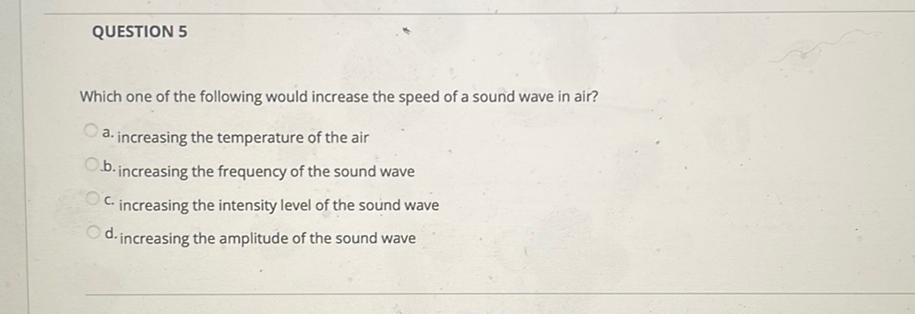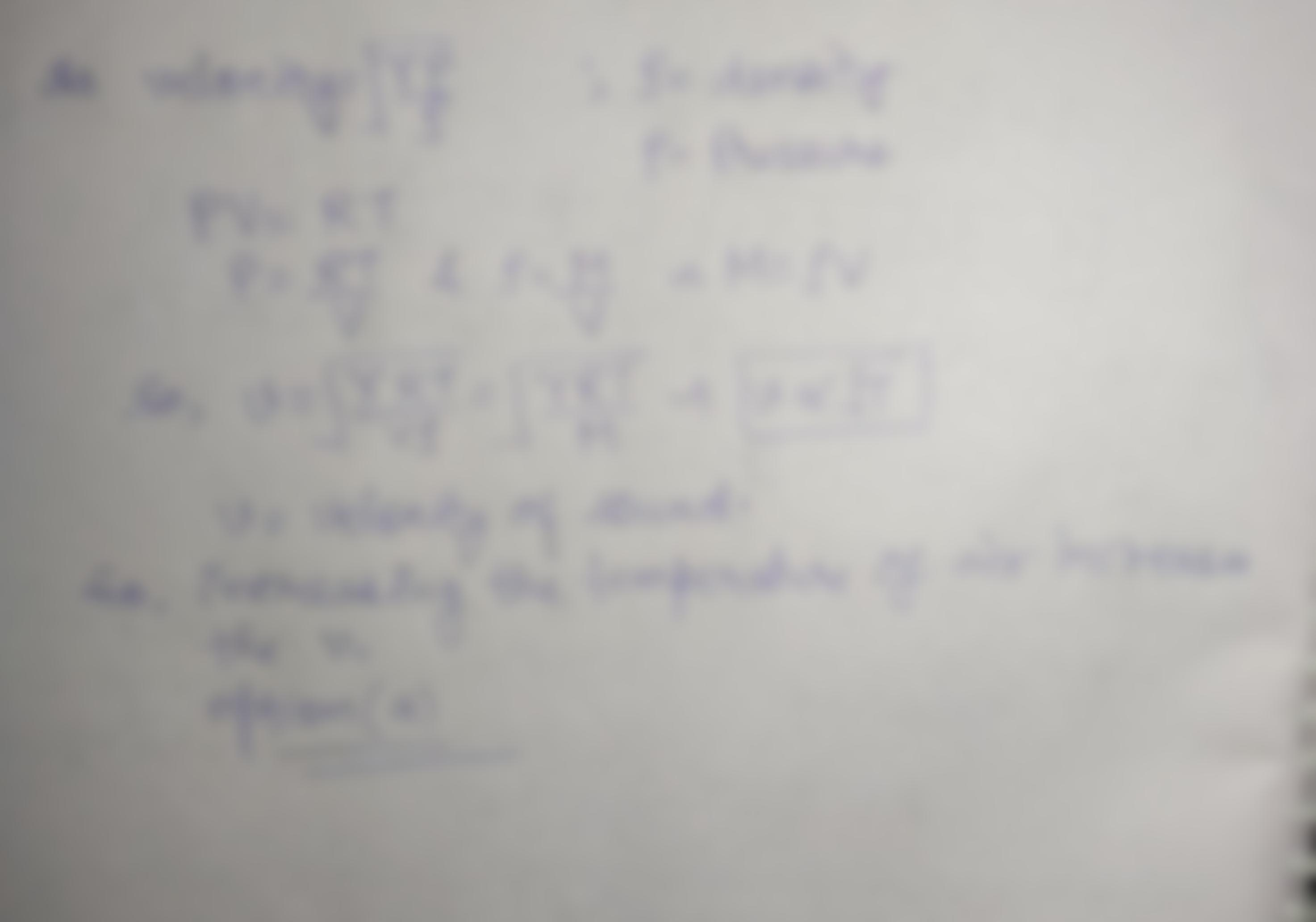Question:

QUESTION 5 Which one of the following would increase the speed of a sound wave in air? a. increasing the temperature of the airQUESTION 5 Which one of the following would increase the speed of a sound wave in air? a. increasing the temperature of the air b.increasing the frequency of the sound wave Cincreasing the intensity level of the sound wave d. increasing the amplitude of the sound wave